Author: Oscar Cronquist Article last updated on October 17, 2019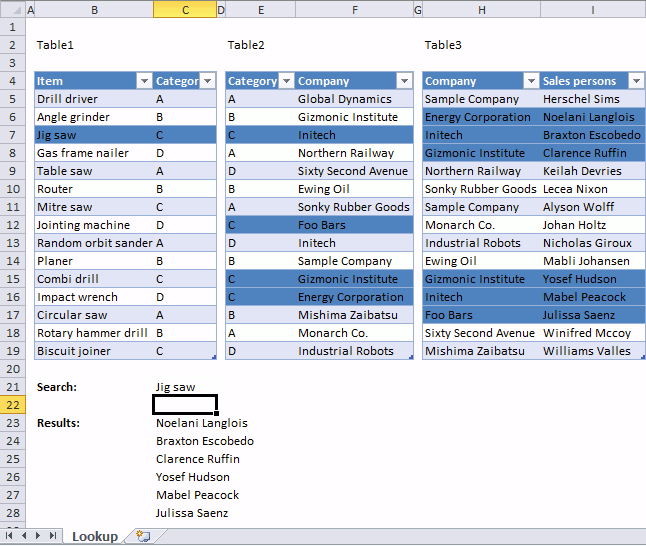This article demonstrates a worksheet that highlights lookups across relational tables. I am using Excel defined Tables, if you add more rows to the Excel tables, the conditional formatting expands automatically.

The Excel user enters a value in cell C21 and the conditional formatting formulas applied to all three Excel Tables highlights values based on the relationship between tables and the search value.

The search value in cell C21 is found in B7, the corresponding value on the same row in Table1 is in cell C7. That value is used to do another lookup in column Category Table2 and four records are found.

Table2 has values in column Company in common with Table3 column Company, this relationship is used to highlight the corresponding values in column "Sales persons".

#### What is a relational table?

In a relational database (Microsoft Access), the data in one table is related to the data in other tables. In general, tables can be related in one of three different ways: one-to-one, one-to-many or many-to-many.  The relationship is used to cross-reference information between tables.
Source: University of Sussex

#### How to apply Conditional Formatting?

1. Select the first Excel Table (cell range B5:C19).
2. Go to tab "Home" on the ribbon.
3. Press with left mouse button on the "Conditional Formatting" button.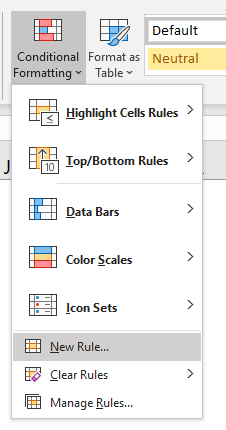4. Press with left mouse button on "New Rule..." and a dialog box appears.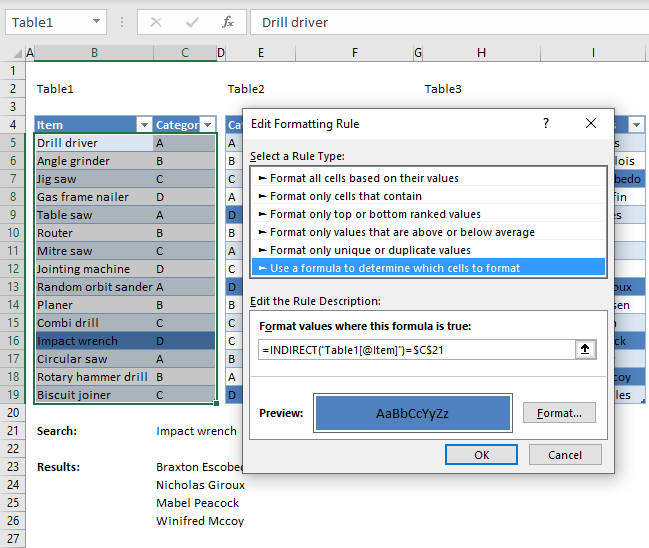5. Press with left mouse button on "Use a formula to determine which cells to format".
6. Type the formula below in "Format values where this formula is true:"
7. Press with left mouse button on "Format" button.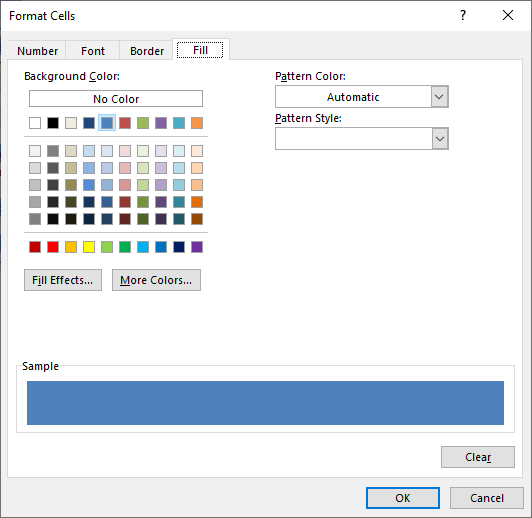8. Go to tab "Fill.
9. Pick a color.
10. Press with left mouse button on OK button.
11. Press with left mouse button on OK button.
12. Repeat steps with the remaining Excel Tables and the corresponding CF formulas described below.

#### Conditional formatting formula applied to Excel Table Table1

=INDIRECT("Table1[@Item]")=\$C\$21

You need to use the INDIRECT function to be able to reference an Excel defined Table in a Conditional Formatting formula. Table1[@Item] is a "structured reference" which is a way to reference Excel Tables, the @ character tells Excel to use only the cell in the same row as the Conditional Formatting formula in column Item.

This allows Excel to compare each value in column Item with the value specified in cell \$C\$21. This cell reference is an absolute cell reference which you can tell by the dollar characters (\$). An absolute cell reference is locked to a specific cell and does not change when the Conditional Formatting formula moves to the next cell.

We do want it to always compare the value in cell C21 with all values in column Item, no matter what.

#### Conditional formatting formula applied to Excel Table Table2

=INDEX(INDIRECT("Table1[Category]"), MATCH(\$C\$21, INDIRECT("Table1[Item]"), 0))=INDIRECT("Table2[@Category]")

### Explaining Conditional Formatting formula in Table2The steps below demonstrate what is calculated by the Conditional Formatting formula in cell E5.

#### Step 1 - Find matching items

The MATCH function returns the relative position of the matching value in column Item Table1.

MATCH(\$C\$21, INDIRECT("Table1[Item]"), 0)

becomes

MATCH("Jig saw", {"Drill driver"; "Angle grinder"; "Jig saw"; "Gas frame nailer"; "Table saw"; "Router"; "Mitre saw"; "Jointing machine"; "Random orbit sander"; "Planer"; "Combi drill"; "Impact wrench"; "Circular saw"; "Rotary hammer drill"; "Biscuit joiner"}, 0)

and returns 3. "Jig saw" is on the third row in column Item Table1.

#### Step 2 - Return the value on the same row from column Category

The INDEX function returns a value from column Category based on the relative row number returned from the MATCH function.

INDEX(INDIRECT("Table1[Category]"), MATCH(\$C\$21, INDIRECT("Table1[Item]"), 0))

becomes

INDEX(INDIRECT("Table1[Category]"), 3)

becomes

INDEX({"A";"B";"C";"D";"A";"B";"C";"D";"A";"B";"C";"D";"A";"B";"C"}, 3)

and returns "C".

#### Step 3 - Compare value in column Category Table 1 with Category Table2

INDEX(INDIRECT("Table1[Category]"), MATCH(\$C\$21, INDIRECT("Table1[Item]"), 0))=INDIRECT("Table2[@Category]")

becomes

"C"=INDIRECT("Table2[@Category]")

becomes

"C"="A"

returns FALSE. Cell E5 in Table2 is not highlgihted.

#### Conditional formatting formula applied to Excel Table Table3

=AND(ISERROR(MATCH(INDIRECT("Table3[@Company]"), IF(ISERROR(MATCH(INDIRECT("Table2[Category]"), IF(\$C\$21=INDIRECT("Table1[Item]"), INDIRECT("Table1[Category]"), ""), 0)), "", INDIRECT("Table2[Company]")), 0)))=FALSE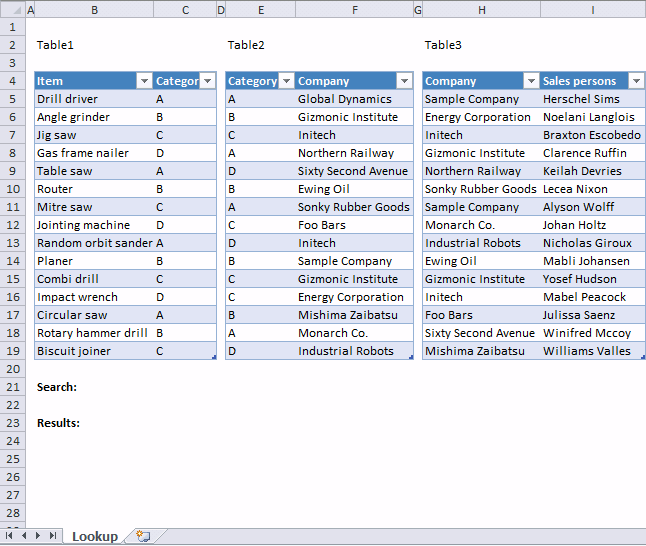The animated image above shows what happens when different search conditions are used.

You can find the array formula in cell C23 here:

Lookups in three related tables and return multiple values

### Get the Excel fileConditional-formatting-in-related-tables.xlsx